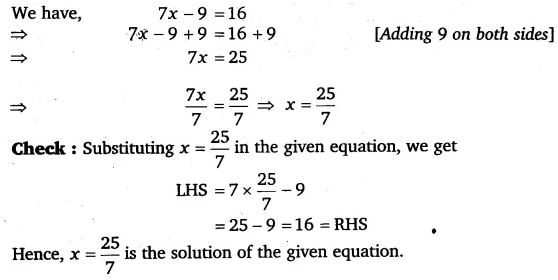# Solve the following equations: 7x – 9 = 16

Solve the following equations:
7x – 9 = 16